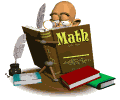• # Math In Focus Parent InformationMath in Focus®(MIF) is a problem solving, hands on curriculum originally taught in Singapore. MIF develops a conceptual understanding of numbers.  This is accomplished by moving from the concrete to pictorial to abstractConcrete learning is introduced through hands-on activities.  These include manipulatives already known to students such as counters, coins and number lines.  Pictorial learning helps students to illustrate the concept with pictures, drawings (bar models) and workbook pages. The abstract stage is the way students practice with numbers and symbols.
• building problem-solving skills and strategies
• using models to solve real-world problems with the four operations
• making and interpreting data from bar graphs
• identifying fractions of a set
• finding angles and identifying lines• understanding area and perimeter of figures*